Courses

# Conductance - Electrochemistry Chemistry Notes | EduRev

## : Conductance - Electrochemistry Chemistry Notes | EduRev

The document Conductance - Electrochemistry Chemistry Notes | EduRev is a part of the Course Physical Chemistry.

CONDUCTANCE

Specific Conductance

Molten electrolytes and the aqueous solutions of electrolytes contain free ions and conduct electricity due to the movement of ions.
We know that Ohm’s law is applicable to metallic conductors as well as electrolytic conductors.
According to Ohm’s law, the resistance of a conductor is directly proportional to the length and is inversely proportional to the area of cross-section of the conductor.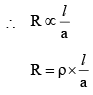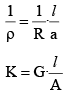where ‘l’ is the distance between the electrodes, ‘a’ is the area of cross-section of electrodes, r is the resistivity of the solution, K is conductivity (specific conductance) of the solution and G is conductance.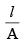is cell constant.

Now, if l = 1 unit of length and a = 1 unit of area, then:

K = G
i.e., K is conductance of the solution which is placed 1 unit length apart between the electrodes of 1 unit area.
Thus, specific conductance is the conductance of a conductor which is observed when it is 1 cm in length and 1 sq. cm in cross-sectional area. In other words, it is the conductance of 1 cc of the electrolyte.
The unit of resistance is ohm (Ω) so unit of conductance will be ohm-1 or Ω-1 and expressing l in cm and a in cm2, the unit of specific conductance are Sm-1 where S stands for Semen.

Molar Conductance (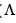m ) :

The conductance of all the ions furnished by one mole of an electrolyte in any solution is termed as its molar conductivity.
Thus, molar conductance is expressed as Molar conductance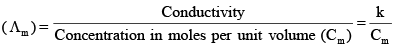Expressing molar conductance in unit of Ω-1cm2mol-1, mathematical expression assumes the form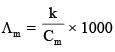Si units ofm is S m2 mol-1

Herem is molar conductance

K is conductance of solution

Cm is concentration of solution in terms of molarity.

Equivalent Conductance : Equivalent conductance is the conducting power of all the ions produced by one g-equivalent i.e. one equivalent of an electrolyte in a  given solution. The equivalent conductance my, therefore, be defined as the conductance which is observed when two sufficiently large electrodes are dipped into solution at unit distance so as the enclose in between them the entire volume of solution containing one equivalent of the electrolyte.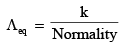Expressingeq in S cm2 equiv-1.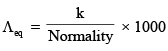Relationship Between Molar and Equivalent Conductivities= n X´eq

Where n = n factor of electrolyte = total charge carried by either of the positive or negative ion.

Variation of Conductance with Dilution

Upon dilution i.e. lowering concentration, specific conductance decrease while equivalent and molar conductances increase. At infinite or almost zero concentration equivalent conductance and molar conductance attain their respective limiting values called equivalent conductance at infinite dilution (∞) or zero concentration (0) and molar conductance at infinite dilution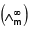or zero concentration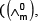, respectively. The increase oform with dilution of a weak electrolyte is attributable to increase of degree of dissociation with dilution resulting into more number of ions in solution. Note that ions are carriers of electricity. The increase ofandm of a strong electrolyte which remains completely ionised at all dilutions, is attributed to increase in the ionic mobilities of ions due decrease in inter-ionic attraction. As dilution approaches unity, the member of ions becomes maximum and henceas well asm approach their respective maximum value. In the case of strong electrolyte, however, the maximum value oform is attained due to the maximum ionic mobilities of the ions since at infinite dilution the dissociation of strong electrolyte is complete and inter-ionic attraction cases to exist completely.
The decrease in K may also be explained in the following way. Upon dilution the number of ions, in the case of weak electrolyte, increase but volume of solution also increases. The increase of volume is in greater proportion than the increase of number of ions resulting into decrease in number of ions per c.c. solution. The specific conductivity being the conductivity of 1 c.c. solution, should obviously decrease.
The variation of molar conductance of a strong electrolyte with concentration is theoretically give by

Debye-Hückel-Onsager equation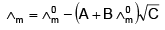Where A and B are the Debye-Hückel constants depending upon nature of the solvent and temperature and C is the molar concentration of solution.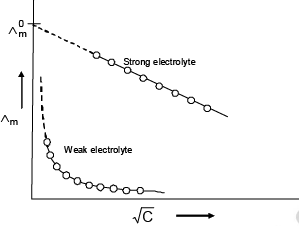Arrhenius Theory of Electrolytic Dissociation for weak Electrolytes: According to the Arrhenius theory of electrolytic dissociation, there exists an equilibrium between the undissociated molecule AB and the ions A+ and B which result from the dissociation of the molecule.
Thus,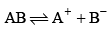This equilibrium is characterized by the equilibrium constant, defined as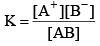If a is the degree of dissociation and c is the initial concentration of the weak electrolyte AB, then concentrations of various species are :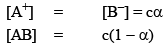Thus K =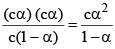Since α << 1, we have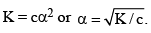The expression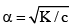is known as the Ostwald dilution law, according to which the degree of dissociation increases as c decreases.  As a is increased, the concentration of the ion A+ and B- are also increased.  Thus, the increase of conductance for a weak electrolyte is primarily due to the increase in the number of ions on dilution.

Debye-Huckel-Onsager Theory Ionic Atmosphere for strong electrolytes
The conductance data of many substances did not conform to Ostwald dilution law. These substance are strong electrolytes which are known to be completely ionized in solution.  The variation of molar conductivity of a dilute solution of a strong electrolyte on dilution is explained in the basis of DebyeHuckel-Onsager theory.  According to this theory, each ion in solution is surrounded by an ionic atmosphere consisting of other ions whose net average charge is opposite to that of the central.  Ion this ionic atmosphere is spherical and symmetrical in nature in the absence of any disturbing factor such as the application of potential across the two electrodes immersed in the solution during the conductance measurements. However, when the potential is applied, the ions start moving towards the respective electrodes and a as a result the ionic atmosphere is distorted which result in a decrease in speed of the ions.  Debye and Huckel showed that these effects are due to two factors, namely,
(a) relation of ionic atmosphere due to an applied potential or symmetry effect and
(b) the electrophoretic effect.

Asymmetry Effect : This arises from the fact that any central ion and is atmosphere are oppositely charged, i.e., when the central ion is positively charged, the atmosphere is negative, and vice versa.  Because of this, the central ion and the atmosphere tend to move in opposite directions as the potential is applied across the electrodes.  Thus, a central positive ion will tend to move towards the cathode while its ionic atmosphere will tend towards the anode. This results in the distortion of spherical and symmetrical nature of the ionic atmosphere as shown in figure.  Consequently, the force exerted by the atmosphere on the central ion is no longer uniform in all directions : it is greater behind the ion than in front of it. Thus, the central ion experiences a retarding force opposite to the direction of its motion with the result that its speed lowered.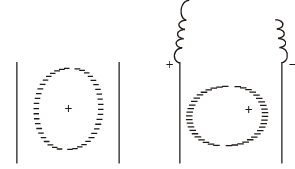Electrophoretic Effect: Ions in solution are generally solvated and when these move, they carry with them the associated solvent molecules. Since cations and anions move in the opposite directions, it is obvious that any particular ion, say the central ion, does not move through a stationary medium but through a medium in which solvent molecules carried by the ions of the atmosphere move in the opposite direction.  Similarly, the ion involved in the ionic atmosphere move in a medium of opposite moving solvent molecules carried by the central ion.  Thus, both type of ions, while moving in solution do not travel through a stationary medium but through a medium which moves in the opposite direction.  These counter-currents make it more difficult for the ion to move through the solution and thus slow down its motion. This is known as the electrophoretic effect.

Onsager expression for strong electrolytes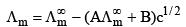The first term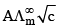is the decrease in molar conductivity due to the asymmetric effect.
The second term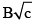is the decrease in molar conductivity due to the electrophoretic effect.

Kohlrausch’s Law of Independent Migration of Ions: At very low concentrations, the molar conductivity of a strong electrolyte tends to reach a limiting value.
This value of molar conductivity is called the molar conductivity at infinite dilution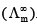. At such high dilutions, the interionic attractions become negligible and each ion migrates independent of the other ions. These observations were used by Kohlrausch to postulate a famous law called Kohlrauschs law of independent ion migration. The Kohlrauschs law in terms of molar conductivity is stated as follows: "At infinite dilution, the molar conductivity of an electrolyte can be expressed as the sum of contributions from its individual ions.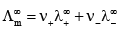where n+ and n- are the numberr of cations and anions per formula unit of electrolyte respectively and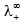and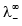are the molar conductivities of the cation and anion at infinite dilution respectively..

Application of Kohlrausch’s Law

(i) Determination of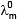of a weak electrolyte

In order to calculate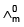of a weak electrolyte say CH3COOH, we determine experimentally Ù0m values of the following three strong electrolysis:

(a) A strong electrolyte containing same cation as in the last electrolyte, say HCl
(b) A strong electrolyte containing same anion as in the test electrolyte, say CH3COONa
(c) A strong electrolyte containing same anion of (a) and cation of (b) i.e. NaCl.  of CH3COOH is the given as(CH3COOH) = (HCl) + (CH3COONa) - (NaCl)

Proof:
(HCl) =                    ......I
(CH3COONa) =      ......II
(NaCl) =                .......III

Adding equation (I) and equation (II) and substracting(III) from them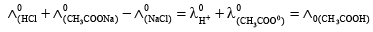(ii) Deter mination of degree of dissociation (α)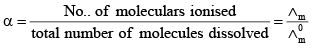(iii) Determination of solubility of sparingly soluble salt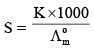S is solubility of sapringly soluble salt.

(iv) Determination of ionic product of water

[H+ ] = [HO-]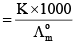K= [H+]2

Kw = ionic product of water

TRANSPORT NUMBER

According to Faraday’s first law of electrolysis, the number of ions discharged at an electrode is proportional to the total quantity passed through the solution, hence it follows that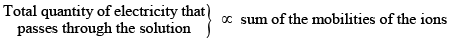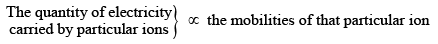The fraction  of the total current carried by each ion is called transport number.  Thus, if u+ is the mobility of cation and u- that of the anion, then

Transport number of the cation,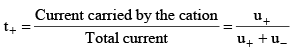Similarly, transport number of the anion,  t = u/(u+ u)
Further, since t+ + t = 1, it follows that if the transport number of one of the ions is known, that of the other can be easily calculated.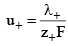Offer running on EduRev: Apply code STAYHOME200 to get INR 200 off on our premium plan EduRev Infinity!

## Physical Chemistry

84 videos|106 docs|31 tests